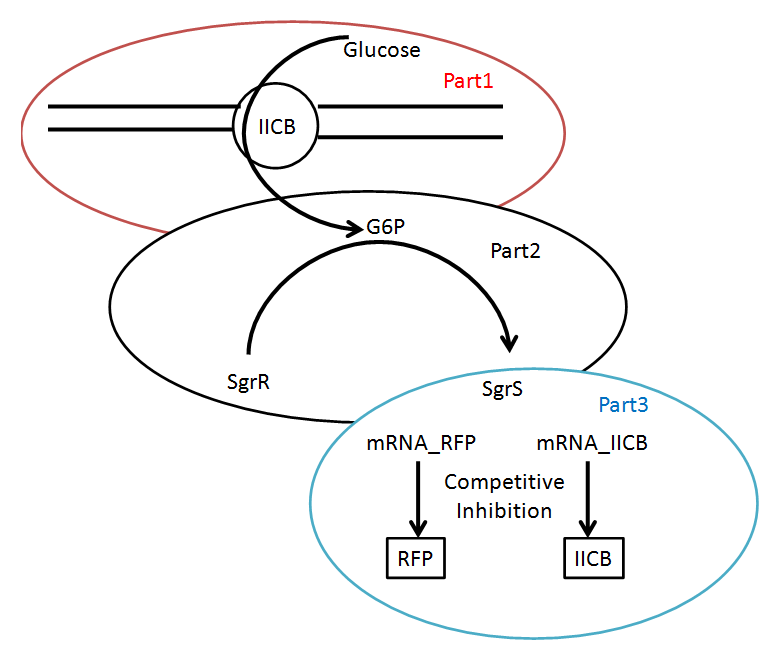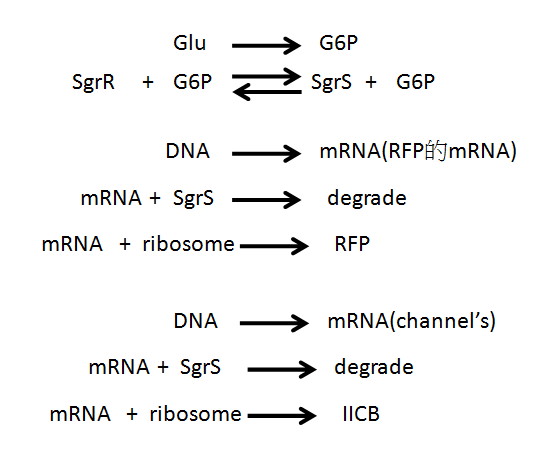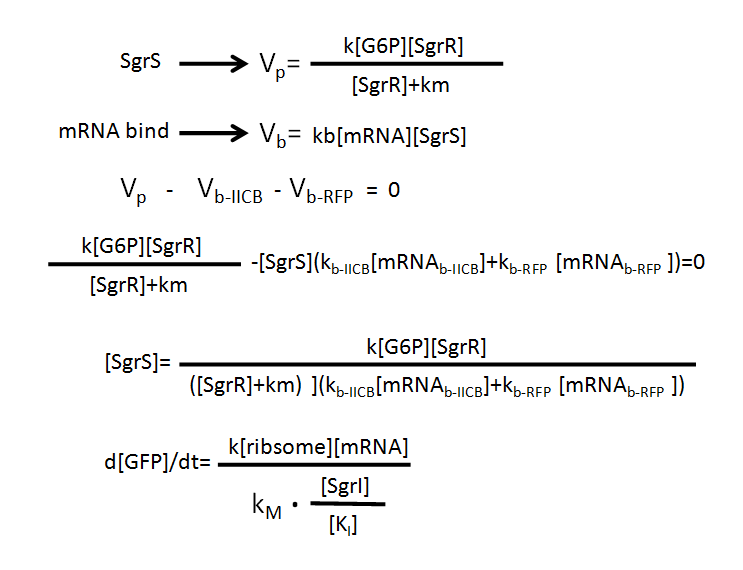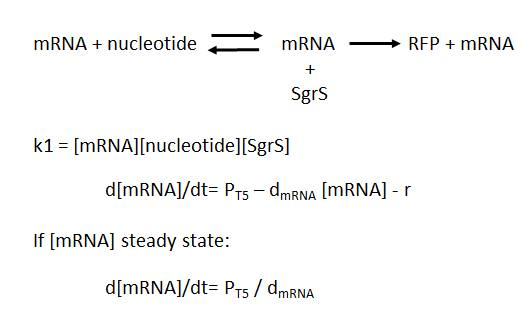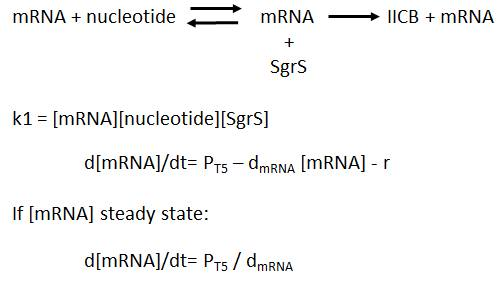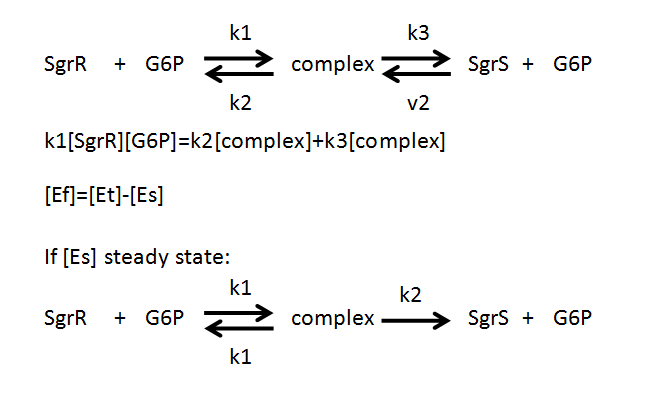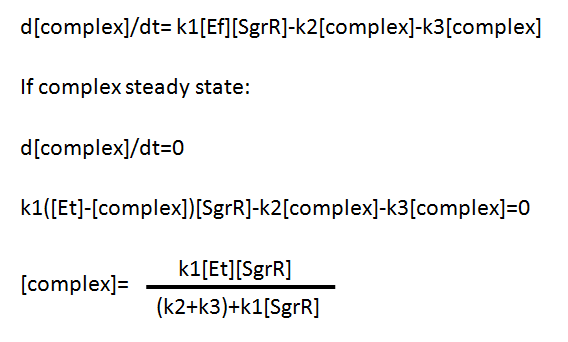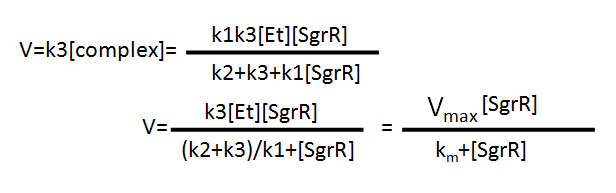# Team:TCU Taiwan/Model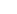We first calculated the rate of accumulation of the G6P. After a few tests, we got the average rate of accumulation. Next we used the MM equation to calculate the rate of production of the SgrS. As the G6P accumulates, SgrS will be synthesised. However, the process would be halted as the mRNA binding process takes place. When the binding rate and the accumulation rate of the G6P is the same, we are able to determine the equilibrium concentration of the SgrS. With the same principle, we are also able to calculate that when the Gloucester is over concentrated, the production of the coloured chromoprotein will come to a halt.